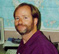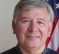## FIR All pass filter with Linear PhaseStarted by 6 years ago9 replieslatest reply 6 years ago1917 views

Hi Everyone

I know most all pass filters are IIR filter and FIR filter with symmetry has a linear phase. Now I would like to design a FIR filter in which it has constant magnitude response and has a linear phase. Does anyone know  the generic structure of all pass FIR filter ? I can easily create linear phase FIR filter but I have a difficulty making it constant magnitude since to be all pass for every zeros I have I should have a pole conjugate and reciprocal of that zero and I know FIR filter does not have a pole. Does this mean there is no all pass linear phase fir filter? The filter I need should have at least three non-zero coefficients.

[ - ]All-pass (box-car) in the frequency domain is the sync function in the time domain.  To make finite, window and assure taps are symmetric and you should have an all-pass, linear-phase FIR filter.

[ - ]You are right the rectangular in time domain is sinc. I will choose a window as well . How can I assure taps are symmetric ? because multiplying with a window gives me certain coefficients and they are not necessary symmetric. Is there any procedural approach for that?

[ - ]Just pick a symmetric window (Rectangular, Barlett, Hamming, Blackman, etc.).

In choosing a window, you may also want to keep in mind that the main and side lobes of the window affect the transition region and ripple, respectively, of the filter.

[ - ]Are you trying to create a filter with an arbitrary phase response that you can select? Or are you trying to theoretically duplicate the phase response given by an "all pass" IIR filter?

[ - ]A FIR filter, with symmetric coefficient structure (thus linear phase) will have some ripple in frequency.  And, a FIR filter, with flat frequency response (thus all pass) will have some ripple in phase - although I don't know how to achieve the latter.

A 3-coefficient FIR filter that's symmetrical in time will have a "DC" or constant component related to the middle coefficient and a sinusoid related to the 1st and 3rd coefficients.  The limit is for the 1st and 3rd coefficients to be zero-valued and, thus, a unit sample.  Then, as the 1st and 3rd coefficients are increased together, the sinusoidal component in the frequency response increases.  Adding coefficient pairs can reduce the sinusoidal component.  With that in mind:

A FIR filter has only zeros and no poles.  An all-pass approximation that is linear phase will have radially inverse (conjugate) pole pairs that are also symmetrical in angular placement (i.e. frequency).  As above, a sinusoidal error in the magnitude response can be constructed with a very simple mathematical expression that yields this zero structure of whatever filter order you choose.

Not your objective but, I've used this method with one additional step to realize all-pass filters that are not symmetrical and not linear phase by taking the square root of the linear phase frequency response expression.  There are multiple solutions.  Each solution is had by removing one of the zeros in a conjugate pair (and its angular match).  If all of the zeros selected are those inside the unit circle then you get a minimum phase filter.  If all of the zeros selected are those outside the unit circle then you get a maximum phase filter.  A *linear* phase filter will have them in conjugate pairs as above.  Perhaps this suggests that a "nearly linear phase - nearly all pass" filter could be had using this method by alternating the zeros in frequency from being inside the unit circle to being outside the unit circle. I've not tried that....

I believe the expressions was:

z = sqrt[(1 + k^(2*N)) + 2 k^N cos((N)*theta)]

If you remove one of the zeros from each zero pair in frequency then that achieves the square root.  So there are lots of choices.

ref: I posted on sci.math on Oct 21, 2002 "Closed form solution?" and received a great response from Robert Israel.

[ - ]We're all trying to figure out what you really want, and then answering the question we imagine you should be asking -- so refining your question for us may be a help.

The only point of an allpass filter is to shift phase.  So you must want to shift phase.  A filter with a linear phase shift gives a constant group delay -- so you must want to time-delay your signal by some constant amount.  Time delaying a signal by an integer number of samples is trivial -- so you must want to time delay your signal by a fractional number of samples.

If your goal is not to time delay your signal by a fractional number of samples, then you are asking the wrong question.  "How do I time delay by a fractional number of samples" is probably a better question to ask.

Two observations immediately spring to mind:

1.  You won't do this with perfectly flat frequency response.  You'll have a trade-off between how perfect the amplitude and phase response are vs. how long the filter is.  Just accept that now.
2. There are probably a bazillion different ways to actually implement this.  If a web search on delaying by a fractional number of samples doesn't help, then don't accept any one way as the best, unless there's some paper that proves that it's optimal -- and then keep in mind that it's only optimal in the sense that the paper solves for.

I would use polyphase filter design techniques.  Assume that you need a delay of M/N samples, where M < N.  I'm assuming that integer delays are irrelevant, and, in fact, that you're going to use the usual DSP dodge of taking the delay of a symmetric FIR filter as zero.

Design a filter that will let you up-sample by a factor of N with sufficient reconstruction for your tastes.  Then one that'll let you down-sample by the exact same factor with sufficient anti-aliasing for your tastes.  Then, in the up-sampled domain, delay the signal by M samples.  Now, go study the polyphase literature to design your overall filter.  Not that I can't tell you exactly how to do this -- I don't know at the moment -- but I expect that it would take less than an hour of my time to actually do it.

The other three alternatives that I can think of are to:

1. Design a boxcar filter, delay it, and do an IFFT.  Boxcar filter designs by means other than Remez exchange are horribly inefficient, though.
2. See if I could expand the Remez exchange algorithm to work with a filter of non-constant phase.  This would probably be beyond me.
3. Find a best-fit filter, in amplitude and phase, using numerical optimization.  This gives you something that you have no deep mathematical understanding of, but which would work.  Folks that came here from comp.dsp would understand why my code would have a comment somewhere saying "RIP Vladimer V.".